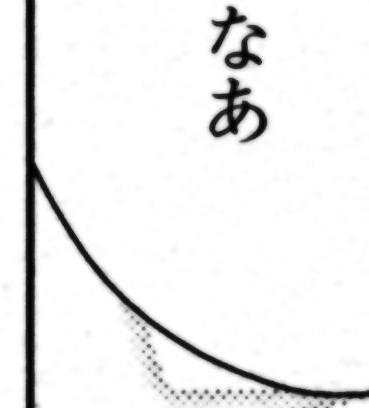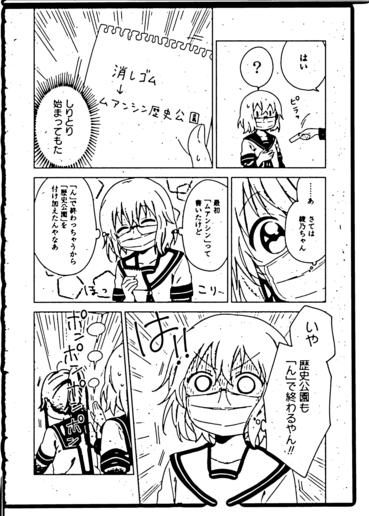## TL;DR

We've looked at automatically rotating pages to line up panel borders. The next step is to crop out the parts that are unevitably from the scanner's lid and not from the page itself. Dory's script will do that.

The script will output a .psd, with a layer containing the page, rotated as best as it could using the result from the previous step (plus one layer for each of the next "probable" guesses"), non-destructively cropped to 7:10 aspect ratio.

## How it works

As we've seen while straightening the page, the page borders are not always parallel to the panel borders. In fact, they rarely do, due to limitations of the printing press. Thus, after rotating the page to straighten up the panel borders, the page borders will almost always be crooked, and most of the times we'll have to crop out part of the page to remove all the content that are not from the page itself. The idea, then, is to find out where the actual page borders are, then fit a largest 7:10 rectangle inside of it.

Most of this is done with OpenCV. We'll be using page 8 from chapter 148, after correcting for panel crookedness, as an example.### Preprocessing

The first step is to preprocess the image to remove as much dust and paper texture as possible. In actual cleaning, we do this with a level, so we'll do the same thing here, just more automatic, and with less worries about losing faint details.

``````# Roughly level the page
black_pt, white_pt = np.percentile(img, (20, 40))
img = skimage.exposure.rescale_intensity(img, in_range=(black_pt, white_pt))``````Then a bilateral (surface blur) filter is applied, to remove some more noise/paper texture, while still keeping edges sharp.

``````# Surface-blur to smooth out noises
img = cv2.bilateralFilter(img, 9, 150, 150)``````With that done, we can then convert the image to binary, using adaptive threshold. This works a little better than absolute threshold around gradient screentones.

``````# Convert to binary image
cv2.THRESH_BINARY, 115, 4)
img = cv2.medianBlur(img, 11)``````### Guessing at the page border

We can extract contours from the binary image. Note how we didn't use any edge detection (e.g. Canny). This is because Canny edge detection has a tendency to produce discontinuous edges around gradients, and we don't want to use any dilation/erosion since those can potentially offset the results by however many pixels we dilate or erode. We don't need to extract features; we just want to find page borders. cv2.RETR_EXTERNAL is used since if a contour is completely contained within another, that one should not be the page border.

``````# Extract contours from edges. The largest of contours will be our page
contours, _ = cv2.findContours(img, cv2.RETR_EXTERNAL, cv2.CHAIN_APPROX_SIMPLE)``````Notice how the contour follows any artwork/panel borders that are black near the page borders. This should be fine for most cases, though, as we'll be using the convex hull of those contours.

To guess which one is the actual page contour, we'll convert those to polygons, and then find out which polygon has the largest convex hull area. The convex hull of that polygon will be our final page border.

``````polys = [cv2.approxPolyDP(contour, 0.0001*img.shape, closed=True)
for contour in contours]
page_poly = max(polys, key=lambda poly: cv2.contourArea(cv2.convexHull(poly)))
convex_poly = cv2.convexHull(page_poly)
poly_area = cv2.contourArea(convex_poly)``````

We also reject any result that's less than 80% of the image area, as a sanity check, to make sure we get something that makes sense as a page border.

``````# Only accept if the page area found is > 80% of image size
if poly_area / img_area < 0.8:
return (0, 0), (img_orig.shape, img_orig.shape) ``````### Finding a decent crop

Once we have a decent page contour polygon, the rest is just feeding this into a Linear Programming solver to find the largest fixed-ratio rectangle that can fit inside that polygon. The objective function would be the height of the crop rectangle (or the width, doesn't matter, since the aspect ratio is fixed). The constraints would be that the 4 corners of the rectangle need to fit within the boundaries of the convex polygon, which can be expressed as a set of linear inequalities describing each of the polygon's edges.

``````poly_matrix = convex_poly.reshape(convex_poly.shape, convex_poly.shape)
X = poly_matrix[:, 0]
Y = poly_matrix[:, 1]
A0 = np.column_stack((np.roll(Y, -1) - Y, X - np.roll(X, -1)))
B0 = np.sum(np.multiply(A0, poly_matrix), axis=1).reshape(A0.shape, 1)

R = 0.7
A1 = (A0 * np.matrix([[0, 0, R, R], [0, 1, 0, 1]]))
A1 = np.reshape(A1, (A1.size, 1))

A0 = np.column_stack((np.repeat(A0, 4, axis=0), A1))
B0 = np.repeat(B0, 4, axis=0)

bounds = [(0, img.shape), (0, img.shape), (0, img.shape)]
solution = linprog(np.matrix('0; 0; -1'), A0, B0, bounds=bounds)
x, y, h = np.rint(solution.x).astype(int)
w = int(np.rint(h*R))``````And that's the result! We can then feed this into a GIMP script to build ourselves a PSD file with the crop without losing any content outside of the cropped area (so that any human editor can come in and fix the crop if needed after-the-fact). Those PSDs can then have the other parts of Photoshop actions applied en masse, automating basically everything in the cleaning pipeline except dusting and redrawing!